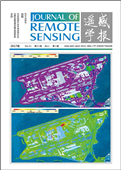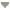出版日期: 2017-01-25点击次数：下载次数：DOI: 10.11834/jrs.201760912017 | Volumn21 | Number 1 上一篇|张立福1 , 陈浩1,2 , 孙雪剑1 , 付东杰1 , 童庆禧1
1. 中国科学院遥感与数字地球研究所, 北京 100101
2. 中国科学院大学, 北京 100049
 收稿日期: 2016-04-07; 修改日期: 2016-09-13; 优先数字出版日期: 2017-01-25 基金项目: 国家自然科学基金（编号：41371362，41371359） 第一作者简介: 张立福(1967-), 男, 研究员, 研究方向为高光谱遥感。E-mail:zhanglf@radi.ac.cn 中图分类号: TP701 文献标识码: A 文章编号: 1007-4619(2017)01-0062-12

# 关键词

Designing spatial-temporal-spectral integrated storage structure of multi-dimensional remote sensing imagesZHANG Lifu1 , CHEN Hao1,2 , SUN Xuejian1 , FU Dongjie1 , TONG Qingxi1
1.The Institute of Remote Sensing and Digital Earth, Chinese Academy of Sciences, Beijing 100101, China
2.University of Chinese Academy of Sciences, Beijing 100049, China

# Abstract

The techniques of satellite remote sensing has been providing massive remote sensing data, however there's no integrated storage structure specially designed for the characters of remote sensing's multi-dimensions. In this context, a method of organizing multi-dimensional remote sensing data is proposed, and an integrated storage structure of SPAtial-Temporal-Spectral (SPATS) multi-dimensional remote sensing images is designed with five multi-dimensional storage format defined:Temporal Sequential in Band (TSB)、Temporal Sequential in Pixel (TSP)、Temporal Interleaved by Band (TIB)、Temporal Interleaved by Pixel (TIP) and Temporal Interleaved by Spectrum (TIS). In addition, Based on this structure, a multi-dimensional data analysis module, namely MDA, is designed, which could implement the SPATS integrated storage of long time-series remote sensing imagery, perform data analysis and display, and build temporal image cubes of a variety of spectral indices, providing a solution of organizing data for the synthesis and characterization of SPATS multi-dimensional remote sending images.

# Key words

remote sensing , integrated storage , multi-dimensional data structure , SPATS , MDA

# 2 SPATS数据结构设计

## 2.1 五种多维存储结构定义

(1) Temporal Sequential in Band (TSB)。如图 1所示，假设多时相遥感数据集包含$T_{1}$$T_{2}$$T_{3}$ 3个时间的影像，每个时间的影像包含3个波段，每个波段4个像元，用4个小方块来表示，图 1下方小方块排列的顺序代表了这种格式中所有像元的存储顺序。立方块前面的数字表示多维数据格式的存储顺序。该数据集包含时间维、光谱维以及空间“行”和“列”共4个维度。

Fig. 1 The storage structure of TSB

TSB存储格式首先将$T_{1}$时间的第一个波段的像元数据按照先行后列的顺序进行存储，然后第一个时间的各个波段顺序存放，最后依据上述规则将多个时间的立方体数据再按时间顺序依次存储。这种存储结构可以表示为

 ${P_{{\rm{TSB}}}} = {F_{{\rm{TSB}}}}\left( 1 \right) + {F_{{\rm{TSB}}}}\left( 2 \right) + \cdots + {F_{{\rm{TSB}}}}\left( T \right)$ (1)

 ${F_{{\rm{TSB}}}}\left( t \right) = {B_{{\rm{TSB}}}}\left( {t,1} \right) + {B_{{\rm{TSB}}}}\left( {t,2} \right) + \cdots + {B_{{\rm{TSB}}}}\left( {t,S} \right)$ (2)

 $\begin{array}{l} \!\!{B_{{\rm{TSB}}}}\left( {t,s} \right) = A\left( {t,s,1 \! : \! C,1} \right) + A\left( {t,s,1 \! : \! C,2} \right) + \cdots + \\ \;\;\;\;\;\;\;\;\;\;\;\;\;\; A\left( {t,s,1 \! : \! C,R} \right) \end{array}$ (3)

(2) Temporal Sequential in Pixel (TSP)。如图 2所示，TSP存储格式首先是将第一个时间的立方体数据按照波段方向采取光谱维优先的原则存储第一个像元的光谱信息，然后各个像元点的光谱再按照“先行后列”的顺序进行存储，最后依据上述规则将多个时间的立方体数据按时间顺序存储，这种存储结构可以表示为

Fig. 2 The storage structure of TSP
 ${P_{{\rm{TSP}}}} = {F_{{\rm{TSP}}}}\left( 1 \right) + {F_{{\rm{TSP}}}}\left( 2 \right) + \cdots + {F_{{\rm{TSP}}}}\left( T \right)$ (4)

 $\begin{array}{l} {F_{{\rm{TSP}}}}\left( t \right) = A\left( {t,1:S,1,1} \right) + A\left( {t,1:S,2,1} \right) + \cdots + \\ A\left( {t,1:S,C,1} \right) + A\left( {t,1:S,1,2} \right) + A\left( {t,1:S,2,2} \right) + \\ \;\;\;\;\;\;\;\;\; \ldots + A\left( {t,1:S,C,2} \right) + \cdots A\left( {t,1:S,1,R} \right) + \\ \;\;\;A\left( {t,1:S,2,R} \right) + \cdots + A\left( {t,1:S,C,R} \right) \end{array}$ (5)

(3) Temporal Interleaved by Band (TIB)。如图 3所示，TIB存储格式首先将第一个波段的第一个时间的像元按照“先行后列”的顺序存储，然后将第一个波段在不同时间上的所有数据按时间顺序组织在一起，最后依据上述规则将所有时间的每个波段依次按照波段的顺序存储，这种存储结构可以表示为

Fig. 3 The storage structure of TIB
 ${P_{{\rm{TIB}}}} = {F_{{\rm{TIB}}}}\left( 1 \right) + {F_{{\rm{TIB}}}}\left( 2 \right) + \ldots + {F_{{\rm{TIB}}}}\left( S \right)$ (6)

 ${F_{{\rm{TIB}}}}\left( s \right) = {B_{{\rm{TIB}}}}\left( {1,s} \right) + {B_{{\rm{TIB}}}}\left( {2,s} \right) + \ldots + {B_{{\rm{TIB}}}}\left( {T,s} \right)$ (7)

 $\begin{array}{l} \!\!{B_{{\rm{TIB}}}}\left( {t,s} \right) = A\left( {t,s,1:C,1} \right) + A\left( {t,s,1:C,2} \right) + \ldots + \\ \;\;\;\;\;\;\;\;\;\;\;\;\; A\left( {t,s,1:C,R} \right) \end{array}$ (8)

(4) Temporal Interleaved by Pixel (TIP)。如图 4所示，TIP首先将第一个波段的第一个像元点在不同时间的数据按时间顺序优先组织在一起，然后所有时间的第一个波段内的像元数据再按“先行后列”的原则顺序存储，最后依据上述规则将所有波段数据按照波段顺序进行存储, 这种存储结构可以表示为

Fig. 4 The storage structure of TIP
 ${P_{{\rm{TIP}}}} = {F_{{\rm{TIP}}}}\left( 1 \right) + {F_{{\rm{TIP}}}}\left( 2 \right) + \ldots + {F_{{\rm{TIP}}}}\left( S \right)$ (9)

 $\begin{array}{l} {F_{{\rm{TIP}}}}\left( s \right) = A\left( {1:T,s,1,1} \right) + A\left( {1:T,s,2,1} \right) + \\ \ldots + A\left( {1:T,s,C,1} \right) + A\left( {1:T,s,1,2} \right) + \\ A\left( {1:T,s,2,2} \right) + \ldots + A\left( {1:T,s,C,2} \right) + \ldots \\ A\left( {1:T,s,1,R} \right) + A\left( {1:T,s,2,R} \right) + \ldots + \\ A\left( {1:T,s,C,R} \right) \end{array}$ (10)

(5) Temporal Interleaved by Spectrum (TIS)。如图 5所示，TIS存储格式首先将第一个像元点所有时间的光谱维数据优先存储，然后依据这一规则将其他像元点按照空间上“先行后列”的原则依次进行存储, 这种存储结构可以表示为

Fig. 5 The storage structure of TIS
 $\begin{array}{l} {P_{{\rm{TSP}}}} = {B_{{\rm{TIS}}}}\left( {1,1} \right) + {B_{{\rm{TIS}}}}\left( {2,1} \right) + \ldots + \\ {B_{{\rm{TIS}}}}\left( {C,1} \right) + {B_{{\rm{TIS}}}}\left( {1,2} \right) + {B_{{\rm{TIS}}}}\left( {2,2} \right) + \ldots + \\ {B_{{\rm{TIS}}}}\left( {C,2} \right) + \ldots + {B_{{\rm{TIS}}}}\left( {1,R} \right) + {B_{{\rm{TIS}}}}\left( {2,R} \right) + \ldots + \\ {B_{{\rm{TIS}}}}\left( {C,R} \right) \end{array}$ (11)

 $\begin{array}{l} {B_{{\rm{TIS}}}}\left( {c,r} \right) = A\left( {1,1:S,c,r} \right) + A\left( {2,1:S,c,r} \right) + \ldots + \\ A\left( {T,1:S,c,r} \right) \end{array}$ (12)

## 2.2 5种结构之间转换

(1) TSB与TSP之间的转换

/* TSP- > TSB */

FOR $t$=0:$T$-1

FOR $s$=0:$S$-1

FOR $i$=0:$R$-1

FOR $j$=0:$C$-1

$P_\rm{TSB}$($t$·$C$·$R$·$S$+$s$·$C$·$R$+$i$·$C$+$j$)=$P$TSP($t$·$C$·$R$·$S$+$i$·$C$·$S$+$j$·$S$+$s$)

END

END

END

END

/* TSB- > TSP */

FOR $t$=0:$T$-1

FOR $i$=0:$R$-1

FOR $j$=0:$C$-1

FOR $s$=0:$S$-1

$P$TSP($t$·$C$·$R$·$S$+$i$·$C$·$S$+$j$·$S$+$s$)=$P_\rm{TSB}$($t$·$C$·$R$·$S$+$s$·$C$·$R$+$i$·$C$+$j$)

END

END

END

(2) TSP与TIS之间的转换

/* TSP- > TIS */

FOR $i$=0:$C$-1

FOR $j$=0:$R$-1

FOR $t$=0:$T$-1

$P$TIS((($i$·$C$+$j$$T+t$$S$(($i$·$C$+$j$$T+t+1)·S-1)= TTSP((t·C·R+i·C+j$$S$:($t$·$C$·$R$+$i$·$C$+$j$+1)·$S$-1)

END

END

END

/* TIS- > TSP */

FOR $t$=0:$T$-1

FOR $i$=0:$C$-1

FOR $j$=0:$R$-1

$P_{\rm{TSP}}$(($t$·$C$·$R$+$i$·$C$+$j$$S:(t·C·R+i·C+j+1)·S-1)= P_{\rm{TIS}}(((i·C+j$$T$+$t$$S((i·C+j$$T$+$t$+1)·$S$-1)

END

END

(3) TSB与TIB之间的转换

/* TSB- > TIB */

FOR $s$=0:$S$-1

FOR $t$=0:$T$-1

$P_\rm{TIB}$(($s$·$T$+$t$$C·R:(s·T+t+1)·C·R-1)= P_\rm{TSB}((t·S+s$$C$·$R$:($t$·$S$+$s$+1)·$C$·$R$-1)

END

END

/* TIB- > TSB */

FOR $s$=0:$S$-1

FOR $t$=0:$T$-1

$P_\rm{TSB}$(($t$·$S$+$s$$C·R:(t·S+s+1)·C·R-1)= P_\rm{TIB}((s·T+t$$C$·$R$:($s$·$T$+$t$+1)·$C$·$R$-1)

END

END

(4) TIB与TIP之间的转换

/* TIB- > TIP */

FOR $s$=0:$S$-1

FOR $i$=0:$R$-1

FOR $j$=0:$C$-1

FOR $t$=0:$T$-1

$P_\rm{TIP}$($s$·$C$·$R$·$T$+$i$·$C$·$T$+$j$·$T$+$t$)=$P_\rm{TIB}$($s$·$C$·$R$·$T$+$t$·$C$·$R$+$i$·$C$+$j$)

END

END

END

END

END

/* TIP->TIB */

FOR $s$=0:$S$-1

FOR $t$=0:$T$-1

FOR $i$=0:$R$-1

FOR $j$=0:$C$-1

$P_{\rm{TIB}}(s·C·R·T+t·C·R+i·C+j)=P_{\rm{TIP}}(s·C·R·T+i·C·T+j·T+t)$

END

END

END

END

(1) TIP与TSB之间的转换。通过TIP↔TIB↔TSB这种顺序依次转换实现。

(2) TIP与TSP之间的转换。通过TIP↔TIB↔TSB↔TSP这种顺序依次转换实现。

(3) TIP与TIS之间的转换。通过TIP↔TIB↔TSB↔TSP↔TIS这种顺序依次转换实现。

(4) TIB与TSP之间的转换。通过TIB↔TSB↔TSP这种顺序依次转换实现。

(5) TIB与TIS之间的转换。通过TIB↔TSB↔TSP↔TIS这种顺序依次转换实现。

(6) TSB与TIS之间的转换。通过TSB↔TSP↔TIS这种顺序依次转换实现。

## 2.3 数据格式分析

(1) TSB格式。从前面格式的存储结构示意图中可以看出，TSB格式将每一个时间的所有波段数据组织在一起，因此这种格式最适合于对一个时间的若干个波段数据进行操作，以及对波段在空间维的处理。TSB格式比较典型应用场景包括：提取一个或多个时间所有波段组成的光谱立方体数据，光谱运算和空间域滤波等。

Fig. 6 Exracting image cube of characteristic parameters

(2) TSP格式。TSP格式的特点是将每个时间影像的光谱数据组织在一起，因此这种格式最适合于对一个时间的光谱数据进行操作。TSP格式比较典型应用场景包括：提取一个像元或者一片区域的光谱曲线，以及对不同时间的影像进行光谱特征化，例如计算光谱斜率和坡向、光谱二值编码、光谱吸收指数、光谱倒数和积分等。

Fig. 7 Spectral integration and continuum removal with TSP

(3) TIB格式。TIB格式的特点是将一个波段所有时间数据组织在一起，因此这种格式最适合于提取某个波段的时间序列立方体数据。TIB格式与TSB不同，前者是将相同波段、不同时间组织在一起，而后者是将相同时间、不同波段数据聚集在一起。TIB格式典型应用场景包括：提取一个波段的时间序列立方体，以及针对某个波段，选择3个时间的数据用于假彩色合成显示。

Fig. 8 Extracting time-series cube for certain band with TIB

(4) TIP格式。TIP格式最大的特点是将图像中像元的时谱数据组织在一起，因此这种格式最适合于对像元的时间谱进行处理与分析。TIP格式比较典型的应用场景包括：提取一个像元或者一片区域在某个波段的时谱曲线，在时间维进行平滑和滤波处理，对时间谱曲线进行拟合，以及进行预测分析等。

Fig. 9 Temporal filter and display with TIP

(5) TIS格式。TIS格式的特点是将每一个像元在整个时间序列上的光谱数据组织在一起，因此这种格式最适合于提取像元光谱曲线的时间序列数据。典型的应用场景是抽取某一个像元在一个时间范围内的所有光谱曲线，然后对这些曲线进行3维可视化，并分析该像元的光谱随着时间变化的情况。

Fig. 10 Extracting time-series dataof spectral curve with TIB and three dimensional display

# 3 多维分析模块构建

## 3.1 文件结构设计

SPATS结构数据由两部分组成：数据文件和头文件。数据文件是实际存储影像数据的文件，影像数据以TSB、TSP、TIP、TIB、和TIS中的一种格式存储在这个文件中，头文件既记录了影像数据本身的信息，包括空间、光谱和时间维的大小、数据存储格式、数据类型，也记录了关于影像数据附加的描述信息，包括坐标投影和仿射变换系数、光谱维和时间维的名称以及文件名称和类型、数据偏移等描述，如图 11。采用这种头文件与数据文件分离的方式使得数据的使用更加灵活，使用者可以利用外部工具打开头文件，查看和修改关于数据文件的信息。设计的头文件后缀名为mdr，数据文件后缀名为mdd。

Fig. 11 The head file of MDD

## 3.2 模块介绍

(1) MDD数据构建及打开。MDD多维数据需要利用经过预处理后的时间序列遥感影像来构建，预处理过程主要包括影像的配准与裁剪，该过程可以由MDA多维分析软件自动完成。预处理过的影像在空间覆盖范围以及像元大小上完全一致，每一个时间的影像是由多个波段组成数据立方体，通常立方体内部存储格式是BSQ或者BIP，每个立方体文件的文件名以时间命名，例如：2002011、2002035、2002043等(表示影像获取的年份以及Day of Year (DOY))，并存放在一个文件夹中。图 12为MDD构建界面，首先指定源数据存放的文件夹路径，同时指定输出文件的文件名、路径，以及构建数据的格式。在确认输入输出之后，构建模块根据文件名自动生成指定格式的MDD数据。

Fig. 12 MDD builder

(2) 格式转换。初始构建MDD数据的格式不一定适用于具体的应用，此时需要进行格式转换，尽可能减少数据提取和分析中的时间。图 13为格式转换界面，首先指定格式转换的输入和输出数据，模块会根据输入数据的头文件自动判断待转换格式；然后指定输出格式，在确定输入输出后便自动完成转换。通过格式转换，在以后相关操作中就能最大限度的减少计算时间。

Fig. 13 Format conversion

(3) 数据提取。数据提取功能支持3种数据的提取，第一种是时谱特征参量的提取，它是首先进行光谱运算然后输出，另外两个分别是时间立方体提取和光谱立方体提取，它们是直接从时-空-谱4维数据中提取3维数据立方体。如图 14界面所示，首先指定待导出数据的路径，然后指定导出数据的空间范围以及类型，如果选择类型为特征参量立方体，首先需要输入特征参量计算表达式，并且指定表达式中各个变量对应的波段，进一步选择提取的时间序列和输出路径，确认之后模块会自动完成提取过程。如果在图 14界面中选择提取类型为光谱立方体，此时选择一个时间和多个待输出的波段，并指定输出路径。再者如果图 14中选择提取类型为时间立方体，与光谱立方体不同的是，此时是选择一个波段以及多个时间。

Fig. 14 Choosing the type of export

(4) 数据列表与显示。如图 15所示，构建或打开MDD数据后会在available data list中出现该数据集的对应信息，包括：波段(bands)、时间(temporal)，以及图像坐标信息(map info)。“temporal dimension”和“spectral dimension”两个选项卡可以来回切换，它们分别表示图像显示时第3个维度(第1、2是平面的2维度)是时间维或光谱维。具体来说，如果选择“temporal dimension”(如图 15(a))，表示第3维为时间，这时需要选择一个波段的一个时间来进行灰度图显示，或者选择这个波段的3个时间来进行假彩色合成显示，在图中“band”位置选择1个波段，然后在“selected time”中选择1个或3个时间(gray scale时选择一个时间，RGB时选择3个时间)，最后加载显示；同理，如果选择“spectral dimension”(如图 15(b))，这时在“time”位置选择1个时间，然后在“selected band”中选择1个或3个波段加载显示。

Fig. 15 The list of available data

## 3.3 处理流程分析

Fig. 16 Flow chart of data processing for applications with MDA multi-dimensional analysis module

Fig. 17 Flow chart of data processing for applications without MDA multi-dimensional analysis module

## 3.4 时谱分类应用

Fig. 18 Flow chart of the classification using time-series data (MDA)

Fig. 19 Flow chart of the classification using time-series data (ENVI)

Table 1 The comparison for processing steps and spending time

 利用ENVI 利用MDA 步骤编号 平均花费时间/s 步骤编号 平均花费时间/s 1 21 1 42 2 15 3 21 2 25 4 15 …… 3 120 51 21 52 10 53 33 54 120 步骤总数 总平均时间 步骤总数 总平均时间 54 1089 3 187

# 4 结论

(1) SPATS多维结构在设计理念上不同于目前现有的组织方式，它不是将一个多维立方体数据通过降维然后用多个低维立方体来表示，而是直接将4维遥感数据当作一个整体，组织成一个4维超立方体数据集。SPATS真正实现了时-空-谱4维遥感数据的一体化组织。

(2) 本文构建的多维分析模块不仅仅是一个时-空-谱4维遥感数据组织的工具，它更是一个以SPATS数据结构为核心，针对多维分析应用的开放平台，无论是空间维分析，还是光谱维或者时间维分析都可以基于这个平台展开。

(3) SPATS数据结构内部定义了5种数据存储格式，分别针对不同的数据分析应用而设计，在具体分析应用的处理速度方面具有优势。

• Galford G L, Mustard J F, Melillo J, Gendrin A, Cerri C C, Cerri C E.2008.Wavelet analysis of MODIS time series to detect expansion and intensification of row-crop agriculture in Brazil. Remote Sensing of Environment, 112 (2): 576–587[DOI: 10.1016/j.rse.2007.05.017]
• Huang C Q, Goward S N, Masek J G, Thomas N, Zhu Z L, Vogelmann J E.2010.An automated approach for reconstructing recent forest disturbance history using dense Landsat time series stacks. Remote Sensing of Environment, 114 (1): 183–198[DOI: 10.1016/j.rse.2009.08.017]
• Irons J R, Dwyer J L, Barsi J A.2012.The next Landsat satellite:the Landsat data continuity mission. Remote Sensing of Environment, 122 : 11–21[DOI: 10.1016/j.rse.2011.08.026]
• Kennedy R E, Yang Z Q, Cohen W B.2010.Detecting trends in forest disturbance and recovery using yearly Landsat time series:1. LandTrendr-Temporal segmentation algorithms. Remote Sensing of Environment, 114 (12): 2897–2910[DOI: 10.1016/j.rse.2010.07.008]
• Margono B A, Turubanova S, Zhuravleva I, Potapov P, Tyukavina A, Baccini A, Goetz S, Hansen M C.2012.Mapping and monitoring deforestation and forest degradation in Sumatra (Indonesia) using Landsat time series data sets from 1990 to 2010. Environmental Research Letters, 7 (3): 034010 [DOI: 10.1088/1748-9326/7/3/034010]
• Mouillot F, Schultz M G, Yue C, Cadule P, Tansey K, Ciais P, Chuvieco E.2014.Ten years of global burned area products from spaceborne remote sensing-A review:analysis of user needs and recommendations for future developments. International Journal of Applied Earth Observation and Geoinformation, 26 : 64–79[DOI: 10.1016/j.jag.2013.05.014]
• Powell S L, Cohen W B, Healey S P, Kennedy R E, Moisen G G, Pierce K B, Ohmann J L.2010.Quantification of live aboveground forest biomass dynamics with Landsat time-series and field inventory data:acomparison of empirical modeling approaches. Remote Sensing of Environment, 114 (5): 1053–1068[DOI: 10.1016/j.rse.2009.12.018]
• Roy D P, Jin Y, Lewis P E, Justice C O.2005.Prototyping a global algorithm for systematic fire-affected area mapping using MODIS time series data. Remote Sensing of Environment, 97 (2): 137–162[DOI: 10.1016/j.rse.2005.04.007]
• Sakamoto T, Van Nguyen N, Kotera A, Ohno H, Ishitsuka N, Yokozawa M.2007.Detecting temporal changes in the extent of annual flooding within the Cambodia and the Vietnamese Mekong Delta from MODIS time-series imagery. Remote Sensing of Environment, 109 (3): 295–313[DOI: 10.1016/j.rse.2007.01.011]
• Sakamoto T, Yokozawa M, Toritani H, Shibayama M, Ishitsuka N, Ohno H.2005.A crop phenology detection method using time-series MODIS data. Remote Sensing of Environment, 96 (3/4): 366–374[DOI: 10.1016/j.rse.2005.03.008]
• Townsend P A, Helmers D P, Kingdon C C, McNeil B E, de Beurs K M, Eshleman K N.2009.Changes in the extent of surface mining and reclamation in the Central Appalachians detected using a 1976-2006 Landsat time series. Remote Sensing of Environment, 113 (1): 62–72[DOI: 10.1016/j.rse.2008.08.012]
• Wulder M A, Masek J G, Cohen W B, Loveland T R, Woodcock C E.2012.Opening the archive:how free data has enabled the science and monitoring promise of Landsat. Remote Sensing of Environment, 122 : 2–10[DOI: 10.1016/j.rse.2012.01.010]